# Compression According to Lempel, Ziv and Welch

## Static and dynamic dictionary techniques

Many data compression methods use dictionaries.  the idea is the following:

• Construct a list of character patterns that occur in the text,
• and encode these patterns as indices of the list.

This procedure is particularly efficient if certain patterns are repeated frequently in the text and this is also taken into account in the coding.  A distinction is made here

• Procedure with static dictionary,
• Procedure with dynamic dictionary.

$\text{(1) Procedure with static dictionary}$

A static dictionary is only useful for very special applications, for example for a file of the following form:

For example, the assignments result in

$$"\boldsymbol{\rm 0}" \hspace{0.05cm} \mapsto \hspace{0.05cm} \boldsymbol{\rm 000000} \hspace{0.05cm},\hspace{0.15cm} ... \hspace{0.15cm},\hspace{0.05cm} "\boldsymbol{\rm 9}" \hspace{0.05cm} \mapsto \hspace{0.05cm} \boldsymbol{\rm 001001} \hspace{0.05cm}, "\hspace{-0.03cm}\_\hspace{-0.03cm}\_\hspace{0.03cm}" \hspace{0.1cm}{\rm (Blank)}\hspace{0.05cm} \mapsto \hspace{0.05cm} \boldsymbol{\rm 001010} \hspace{0.05cm},$$
$$"\hspace{-0.01cm}.\hspace{-0.01cm}" \hspace{0.1cm}{\rm (Punkt)}\hspace{0.05cm} \mapsto \hspace{0.05cm} \boldsymbol{\rm 001011} \hspace{0.05cm}, "\hspace{-0.01cm},\hspace{-0.01cm}" \hspace{0.1cm}{\rm (Komma)}\hspace{0.05cm} \mapsto \hspace{0.05cm} \boldsymbol{\rm 001011} \hspace{0.05cm}, " {\rm end\hspace{-0.1cm}-\hspace{-0.1cm}of\hspace{-0.1cm}-\hspace{-0.1cm}line}\hspace{0.05cm} \mapsto \hspace{0.05cm} \boldsymbol{\rm 001101} \hspace{0.05cm},$$
$$"\boldsymbol{\rm A}" \hspace{0.05cm} \mapsto \hspace{0.05cm} \boldsymbol{\rm 100000} \hspace{0.05cm},\hspace{0.15cm} ... \hspace{0.15cm},\hspace{0.05cm} "\boldsymbol{\rm E}" \hspace{0.05cm} \mapsto \hspace{0.05cm} \boldsymbol{\rm 100100} \hspace{0.05cm}, \hspace{0.15cm} ... \hspace{0.15cm},\hspace{0.05cm} "\boldsymbol{\rm L}" \hspace{0.05cm} \mapsto \hspace{0.05cm} \boldsymbol{\rm 101011} \hspace{0.05cm},\hspace{0.15cm}"\boldsymbol{\rm M}" \hspace{0.05cm} \mapsto \hspace{0.05cm} \boldsymbol{\rm 101100} \hspace{0.05cm},$$
$$"\boldsymbol{\rm O}" \hspace{0.05cm} \mapsto \hspace{0.05cm} \boldsymbol{\rm 101110} \hspace{0.05cm},\hspace{0.15cm} ... \hspace{0.15cm},\hspace{0.05cm} "\boldsymbol{\rm U}" \hspace{0.05cm} \mapsto \hspace{0.05cm} \boldsymbol{\rm 110100} \hspace{0.05cm}, "\boldsymbol{\rm Name\hspace{-0.1cm}:\hspace{-0.05cm}\_\hspace{-0.03cm}\_}" \hspace{0.05cm} \mapsto \hspace{0.05cm} \boldsymbol{\rm 010000} \hspace{0.05cm},\hspace{0.05cm}$$
$$"\boldsymbol{\rm ,\_\hspace{-0.03cm}\_Vorname\hspace{-0.1cm}:\hspace{-0.05cm}\_\hspace{-0.03cm}\_}" \hspace{0.05cm} \mapsto \hspace{0.05cm} \boldsymbol{\rm 010001} \hspace{0.05cm},\hspace{0.05cm} "\boldsymbol{\rm ,\_\hspace{-0.03cm}\_Wohnort\hspace{-0.1cm}:\hspace{-0.05cm}\_\hspace{-0.03cm}\_}" \hspace{0.05cm} \mapsto \hspace{0.05cm} \boldsymbol{\rm 010010} \hspace{0.05cm},\hspace{0.15cm} ... \hspace{0.15cm}$$

for the first line of the above text, binary source coded with six bits per character:

$$\boldsymbol{010000} \hspace{0.15cm}\boldsymbol{100000} \hspace{0.15cm}\boldsymbol{100001} \hspace{0.15cm}\boldsymbol{100100} \hspace{0.15cm}\boldsymbol{101011} \hspace{0.3cm} \Rightarrow \hspace{0.3cm} \boldsymbol{(\rm Name\hspace{-0.1cm}:\hspace{-0.05cm}\_\hspace{-0.03cm}\_) \hspace{0.05cm}(A)\hspace{0.05cm}(B)\hspace{0.05cm}(E)\hspace{0.05cm}(L)}$$
$$\boldsymbol{010001} \hspace{0.15cm}\boldsymbol{101011}\hspace{0.15cm} \boldsymbol{100100} \hspace{0.15cm}\boldsymbol{101110} \hspace{0.3cm} \Rightarrow \hspace{0.3cm} \boldsymbol{(,\hspace{-0.05cm}\_\hspace{-0.03cm}\_\rm Vorname\hspace{-0.1cm}:\hspace{-0.05cm}\_\hspace{-0.03cm}\_) \hspace{0.05cm}(L)\hspace{0.05cm}(E)\hspace{0.05cm}(O)}$$
$$\boldsymbol{010010} \hspace{0.15cm}\boldsymbol{110100} \hspace{0.15cm}\boldsymbol{101011} \hspace{0.15cm}\boldsymbol{101100} \hspace{0.3cm}\Rightarrow \hspace{0.3cm} \boldsymbol{(,\hspace{-0.05cm}\_\hspace{-0.03cm}\_\rm Wohnort\hspace{-0.1cm}:\hspace{-0.05cm}\_\hspace{-0.03cm}\_) \hspace{0.05cm}(U)\hspace{0.05cm}(L)\hspace{0.05cm}(M)} \hspace{0.05cm}$$
$$\boldsymbol{001101} \hspace{0.3cm}\Rightarrow \hspace{0.3cm} ({\rm end\hspace{-0.1cm}-\hspace{-0.1cm}of\hspace{-0.1cm}-\hspace{-0.1cm}line}) \hspace{0.05cm}$$

$\text{Conclusion:}$  In this specific application the first line can be displayed with  $14 \cdot 6 = 84$  Bit.

• In contrast, conventional binary coding would require  $39 \cdot 7 = 273$  bits since:
• Because of the lowercase letters in the text, six bits per character are not sufficient here.
• For the entire text, this results in  $103 \cdot 6 = 618$  Bit versus  $196 \cdot 7 = 1372$  Bit.
• However, the code table must also be known to the recipient.

$\text{(2) Procedure with dynamic dictionary}$

Nevertheless, all relevant compression methods do not work with static dictionaries, but with dynamic dictionaries, which are created successively only during the coding:

• Such procedures are flexible and do not have to be adapted to the application   One speaks of universal source coding procedures.
• A single pass is sufficient, whereas with a static dictionary the file must first be analyzed before the encoding process.
• At the sink, the dynamic dictionary is generated in the same way as for the source .  this eliminates the need to transfer the dictionary.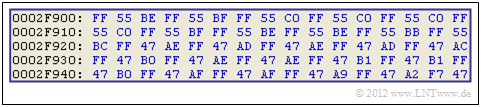Extract from the hexdump of a natural image in BMP format

$\text{Example 1:}$  The graphic shows a small section of  $80$  Byte of a  BMP-File  in hexadecimal representation.  It is the uncompressed representation of a natural picture.

• You can see that in this small section of a landscape image the bytes  $\rm FF$,  $\rm 55$  and  $\rm 47$  occur very frequently.
• Data compression is therefore promising.
• But since other parts of the  $\text{4 MByte}$ file or other byte combinations dominate in other image contents, the use of a static dictionary would not be appropriate here.

$\text{Example 2:}$  For an artificially created graphic, for example a form, you could work with a static dictionary.

We are looking at a b/w image with  $27 × 27$  pixels, where the mapping "black"   ⇒   0  and "white"   ⇒   1  has been agreed upon.

• At the top (black marker) each line is described by  $27$  zeros.
• In the middle (blue marking), three zeros and three ones always alternate.
• At the bottom (red mark), each line is delimited by  $25$  ones by two zeros.

## LZ77 - the basic form of the Lempel-Ziv-algorithms

The most important procedures for data compression with a dynamic dictionary go back to  Abraham Lempel  and  Jacob Ziv  zurück.  The entire Lempel-Ziv family  (in the following we will use for this briefly:   LZ procedure)  can be characterized as follows

• Lempel-Ziv methods use the fact that often whole words, or at least parts of them, occur several times in a text.  One collects all word fragments, which are also called  phrases  in a sufficiently large dictionary.
• Contrary to the entropy coding developed before (by Shannon and Huffman), the frequency of single characters or character strings is not the basis of the compression here, so that the LZ procedures can be applied even without knowledge of the source statistics.
• LZ compression accordingly manages with a single pass and also the source symbol range  $M$  and the symbol set  $\{q_μ\}$  with  $μ = 1$, ... , $M$  does not have to be known.  This is called Universal Source Coding.

We first look at the Lempel-Ziv algorithm in its original form from 1977, known as  LZ77:

• This works with a window that is successively moved over the text  one also speaks of a  sliding window.
• The window size  $G$  is an important parameter that decisively influences the compression result.

The graphic shows an example of the  sliding windows.  This is divided into

• the preview buffer  $($blue background),  and
• the search buffer  $($red background, with the positions  $P = 0$, ... , $7$   ⇒   window size  $G = 8)$.

The edited text consists of the four words  MissMission'Mississippi'  and  Mistral', each separated by a hyphen.  At the time in question the preview buffer contains  Mississi.

• Search now in the search buffer for the best match   ⇒   the string with the maximum match length  $L$.  This is the result for the position  $P = 7$  and the length  $L = 5$  to  Missi.
• This step is then expressed by the triple  $(7, 5,$ s$)$  expressed   ⇒   general  $(P, \ L, \ Z)$, where  $Z =$ s  specifies the character that no longer matches the string found in the search buffer.
• At the end the window is moved by  $L + 1 = 6$  character is moved to the right.  In the preview buffer there is now  sippi-Mi,  in the search buffer  n-Missis'  and the encoding gives the triple  $(2, 2,$  p$)$.

In the following example the LZ77-coding algorithms are described in more detail.  The decoding runs in a similar way.

$\text{Example 3:}$  We consider the LZ77 encoding of the string  ABABCBCBAABCABe  according to the following graphic.  The input sequence has the length $N = 15$.

Further is assumed:

• For the characters apply  $Z ∈ \{$ ABCe $\}$,  where  e  corresponds to the  end-of-file  (end of the input string)
• The size of the preview and search buffer are  $G = 4$   ⇒   Position  $P ∈ {0, 1, 2, 3}$.

Display of the encoding process:

Step 1 and 2:   The characters  A  and  B  are encoded by the triple  $(0, 0,$ A$)$  and  $(0, 0,$ B$)$, because they are not yet stored in the search buffer.   Then move the Sliding Window by 1.

step 3:   AB  is masked by the search buffer and at the same time the still unknown character  C  is appended.  After that the Sliding Window is moved three positions to the right.

Step 4:   This shows that the search string  BCB  may also end in the preview buffer.  Now the window can be moved four positions to the right.

Step 5:   Only  A  is found in the search buffer and  B  is dropped.  If the search buffer is larger, however,  ABC  could be masked together.  For this purpose  $G ≥ must be 7$ .

Step 6:   Likewise, the character  C  must be coded separately due to the buffer being too small.  But since  CA  hasn't occurred before,  would not improve the compression here  $G = 7$

Step 7:   With the consideration of the end-of-file  (e)  together with  AB  from the search buffer, the encoding process is finished.

Before transmission, the specified triples must of course be binary coded.  In this example you need

• the position  $P ∈ \{0, 1, 2, 3\}$  two Bit  (yellow background in the table above),
• the copy length  $L$  three bits  (green background), so that one could also  $L = 7$  still be displayed,
• all characters are two bits  (white background),  for example  A00B01C10e ("end-of-file") → 11.

Thus the LZ77 output sequence has a length of  $7 - 7 = 49$  bit, while the input sequence only needed  $15 - 2 = 30$  bit.

$\text{Conclusion:}$  A Lempel-Ziv compression only makes sense with large files !

## The Lempel-Ziv-Variant LZ78

The LZ77-algorithm produces very inefficient output if more frequent strings are repeated only with a larger distance.  Such repetitions can often not be recognized due to the limited buffer size  $G$  des  Sliding Window .

Lempel and Ziv corrected this shortcoming already one year after the release of the first version LZ77:

• The algorithm LZ78 uses a global dictionary for compression instead of the local dictionary (search buffer).
• The size of the dictionary allows efficient compression of phrases that have been used for a long time before.

$\text{Example 4:}$  To explain the LZ78 algorithm we consider the same sequence  ABABCBCBAABCABe  as for the LZ77-$\text{Example 3}$.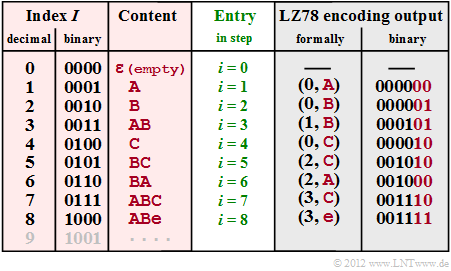Generation of the dictionary and output at LZ78
• The graphic shows  (with red background)  the dictionary with index  $I$  (in decimal and binary representation, column 1 and 2)  and the corresponding content (column 3), which is entered for coding step  $i$  (column 4).

• For LZ78 both encoding and decoding are always  $i = I$.

• In column 5 you find the formalized code output  $($Index  $I$,  new character  $Z)$.

• In column 6 the corresponding binary coding is given with four bits for the index and the same character assignment  A00B01C10e ("end-of-file") → 11  as in  $\text{Example 3}$.

• At the beginning  (step $\underline{i = 0}$)  the dictionary is  (WB)  empty except for the entry  ε  $($empty character, not to be confused with the space character, which is not used here$)$  with index  $I = 0$.
• In the step  $\underline{i = 1}$  there is no usable entry in the dictionary yet, and it becomes  (0,  A)  output  (A  follows  ε).   In the dictionary, the entry  A  follows in line  $I = 1$  (abbreviated  1: A).
• The procedure in the second step  ($\underline{i = 2}$).  The output is  (0,  B)  and the dictionary entry is  2: B .
• As the entry  $\underline{i = 3}$  is already found in step  1: A , the characters  AB  can be coded together by  (1, B)  and the new dictionary entry  3: AB  is made.
• After coding and insertion of the new character  C  in step  $\underline{i = 4}$  the pair of characters  BC  is coded together   ⇒   (2, C) and entered into the dictionary  5: BC  in step  $\underline{i = 5}$ .
• In step  $\underline{i = 6}$  two characters are treated together with  '6: BA  and in the last two steps three each, namely  7: ABC  and  8: ABe.
• The output  (3, C)  in step  $\underline{i = 7}$  stands for  "WB(3) + C = ABC   and the output  (3, e)  in step  $\underline{i = 8}$  for  ABe.

In this  $\text{Example 4}$  the LZ78 code symbol sequence thus consists of  $8 - 6 = 48$  Bit.  The result is comparable to the LZ77-$\text{Example 3}$  $(49$ Bit$)$.

$\text{Conclusion:}$  Details and improvements of LZ78 will be omitted here.  Here we refer to the  LZW-Algorithm, which will be described on the following pages.  Only this much will be said now:

• The index  $I$  is uniformly represented here with four bits, whereby the dictionary is limited to  $16$  entries.  By a variable number of bits  for the index one can bypass this limitation.  At the same time one gets a better compression factor.
• The dictionary does not have to be transmitted with all LZ variants, but is generated with the decoder in exactly the same way as on the coder side.  The decoding is also done with LZ78, but not with LZW, in the same way as the coding.
• All LZ procedures are asymptotically optimal, i.e., for infinitely long sequences the mean code word length  $L_{\rm M}$  per source symbol is equal to the source entropy  $H$ .
• For short sequences, however, the deviation is considerable.  More about this at  end of chapter.

## The Lempel-Ziv-Welch algorithm

The most common variant of Lempel-Ziv compression used today was designed by  Terry Welch  and published in 1983.  In the following we refer to it as the  Lempel-Ziv-Welch-Algorithm, abbreviated as "LZW".   Just as LZ78 has slight advantages over LZ77  (as expected, why else would the algorithm have been modified?),  LZW also has more advantages than disadvantages compared to LZ78.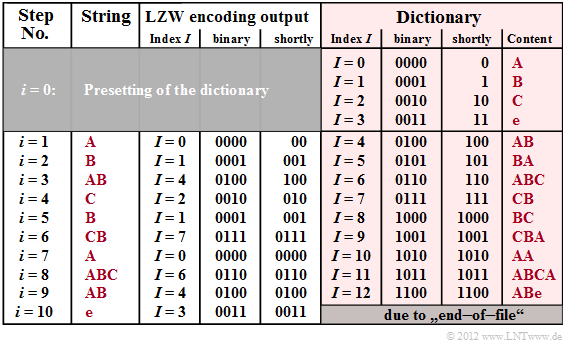LZW encoding of the sequence  'ABABCBCBAABCABe

The graphic shows the coder output for the exemplary input sequence  ABABCBCBAABCABe.  On the right is the dictionary (highlighted in red), which is successively generated during LZW encoding.  The differences to LZ78 can be seen in comparison to the graphic on the last page, namely

• For LZW, all characters occurring in the text are already entered at the beginning of  $(i = 0)$  and assigned to a binary sequence, in the example with the indices  $I = 0$, ... ,  $I = 3$.  This also means that LZW requires some knowledge of the message source, whereas LZ78 is a "true universal encoding".
• For LZW, only the dictionary index  $I$  is transmitted for each encoding step  $i$  while for LZ78 the output is the combination  $(I$,  $Z)$, where $Z$  denotes the current new character.   Due to the absence of  $Z$  in the code output, LZW decoding is more complicated than with LZ78, as described on page  Decoding of LZW–Algorithm .

$\text{Example 5:}$  For this exemplary LZW encoding, as with "LZ77" and "LZ78" again the input sequence  ABABCBCBAABCABe  is assumed.  So the following description refers to the above graphic.

Step i = 0 (default):   The allowed characters  ABC  and  e  ("end-of-file") are entered into the dictionary and assigned to the indices  $I = 0$, ... , $I = 3$ .

Step i = 1:   A  is coded by the decimal index  $I = 0$  and its binary representation  0000  is transmitted.   Then the combination of the current character  A  and the following character  B  of the input sequence is stored in the dictionary under the index  $I = 4$ .

step i = 2:   representation of  B  by index  $I = 1$  or.  0001  (binary) as well as dictionary entry of  BA  placed under index  $I = 5$.

Step i = 3:   Because of the entry  AB  at time  $i = 1$  the index to be transmitted is  $I = 4$  (binary: 0100).  New dictionary entry of  ABC  under  $I = 6$.

Step i = 8:   Here the characters  ABC  are represented together by the index  $I = 6$  (binary: 0110)  and the entry for  ABCA  is made.

With the encoding of  e  (EOF mark) the encoding process is finished after ten steps.  With LZ78 only eight steps were needed.  But it has to be considered:

• The LZW algorithm needs only  $10 \cdot 4 = 40$  Bit versus the  $8 \cdot 6 = 48$  Bit for LZ78.  Provided that for this simple calculation, four bits each are needed for index representation.
• LZW as well as LZ78 require less bits  $($namely   $34$  or   $42)$, if one considers that for the step  $i = 1$  the index has to be coded with two bits only  $(I ≤ 3)$  and for  $2 ≤ i ≤ 5$  three bits are sufficient  $(I ≤ 7)$.

The following pages describe in detail the variable bit count for index representation and the decoding of LZ78- and LZW-encoded binary sequences.

## Lempel-Ziv-Coding with variable index bit length

For reasons of a most compact representation, we now consider only binary sources with the value set  $\{$A, B$\}$.  The terminating character  end-of-file  is also not considered.

We demonstrate the LZW coding by means of a screenshot of our interactive Flash module  Lempel-Ziv-Welch–Algorithms.

• In the first coding step  $(i = 1)$  A   ⇒  0.  Afterwards the entry with index  $I = 2$  and  content  AB.
• As there are only two entries in step  $i = 1$  in the dictionary (  A  and  B ) one bit is sufficient.   On the other hand, for step  $i = 2$  and  $i = 3$  for  B  ⇒  01  and   A  ⇒  00  two bits are needed in each case.
• Starting on  $i = 4$ , the index representation is done with three bits, then from   $i = 8$  with four bits and from  $i = 16$  with five bits.  A simple algorithm for the respective index bit number  $L(i)$  can be derived.
• Let's finally consider the coding step  $i = 18$.  Here, the sequence  ABABB marked in red, which was entered into the dictionary at time  $i = 11$  at time  $($Index  $I = 13$ ⇒ 1101$)$  is edited.   However, the coder output is now  01101 because of  $i ≥ 16$  (green mark at the coder output).

The statements also apply to  LZ78.  That is:   With the LZ78 a variable index bit length results in the same improvement as with the LZW.

## Decoding of the LZW algorithm

The decoder now displays the decoded output on the  last page  as input sequence.  The graphic shows that it is possible to uniquely decode this sequence even with variable index bit lengths. Please note:

• The decoder knows that in the first coding step  $(i = 1)$  the index  $I$ was coded with only one bit, in the steps  $i = 2$  and  $i = 3$  with two bit, from   $i = 4$  with three bit, from  $i = 8$  with four bit, and so on.
• The decoder generates the same dictionary as the coder, but the dictionary entries are made one time step later.

• At step  $\underline{i = 1}$  the adjacent symbol  0  is decoded as  A .   Likewise, the following results for step  $\underline{i = 2}$  from the preassignment of the dictionary and the two-bit representation agreed upon for this:   01   ⇒   B.
• The entry of the line  $\underline{I = 2}$  $($content:   AB$)$  of the dictionary is therefore only made at the step  $\underline{i = 2}$, while at the  Coding process  this could already be done at the end of step  $i = 1$ .
• Let us now consider the decoding for  $\underline{i = 4}$.   The index  $\underline{I = 2}$  returns the decoding result  010   ⇒   AB  and in the next step  $(\underline{i = 5})$  the dictionary line  $\underline{I = 5}$  will be filled with  ABA .
• This time difference with respect to the dictionary entries can lead to decoding problems.  For example, for step  $\underline{i = 7}$  there is no dictionary entry with index  $\underline{I= 7}$.
• What is to do in such a case as  $(\underline{I = i})$?  In this case you take the result of the previous decoding step  $($here:   BA  for  $\underline{i = 6})$  and append the first character of this sequence at the end again.   This gives the decoding result for  $\underline{i = 7}$  to  111   ⇒   BAB.
• Naturally, it is unsatisfactory to specify only one recipe.  In the  Exercise 2.4Z  you should justify the procedure demonstrated here.  We refer to the sample solution for this exercise.

With LZ78 decoding, the problem described here does not occur because not only the index  $I$ but also the current character  $Z$  is included in the encoding result and is transmitted.

## Remaining redundancy as a measure for the efficiency of encoding methods

For the rest of this chapter we assume the following prerequisites:

• The  symbol range  the source  $($or in the transmission sense:   the number of stages)  sei  $M$, where  $M$  represents a power of two   ⇒   $M = 2, \ 4, \ 8, \ 16$, ....
• The source entropy is  $H$.  If there are no statistical bonds between the symbols and if they are equally probable, then  $H = H_0$, where  $H_0 = \log_2 \ M$  indicates the decision content.  Otherwise, $H < H_0$ applies.
• A symbol sequence of length  $N$  is source-coded and returns a binary code sequence of length  $L$.  For the time being we do not make any statement about the type of source coding.

According to the  Source Encoding Theorem  the mean code word length  $L_{\rm M}$  must be greater than or equal to the source entropy  $H$  (in bit/source symbol).  This means

• for the total length of the source-encoded binary sequence:
$$L \ge N \cdot H \hspace{0.05cm},$$
• for the relative redundancy of the code sequence, in the following called  Rest Redundancy:
$$r = \frac{L - N \cdot H}{L} \hspace{0.05cm}.$$

$\text{Example 6:}$  If there were a  perfect source encoding for a redundancy-free binary source symbol sequence  $(M = 2,\ p_{\rm A} = p_{\rm B} = 0.5$,  without statistical bonds$)$  of length  $N = 10000$, the code sequence would have length  $L = 10000$.

Consequence:   If in a code the result  $L = N$  is never possible, then this code is called  not–perfect.

• Lempel-Ziv is not suitable for this redundancy-free message source.  It will always be  $L > N$    You can also put it quite succinctly like this:   The perfect source encoding here is "no encoding at all".
• A redundant binary source with  $p_{\rm A} = 0.89$,  $p_{\rm B} = 0.11$   ⇒   $H = 0.5$  could be represented with a perfect source encoding with $L = 5000$  bit, without being able to say what this perfect source encoding looks like.
• For a quaternary source,  $H > 1 \ \rm (bit/source symbol)$  is possible, so that even with perfect source encoding there will always be  $L > N$ .  If the source is redundancy-free  (no bonds, all  $M$  symbols equally probable), it has entropy  $H= 2 \ \rm (bit/source symbol)$.

For all these examples of perfect source encoding, the relative redundancy of the code sequence (residual redundancy) is  $r = 0$. That is:   The zeros and ones are equally probable and there are no statistical bonds between the individual binary symbols.

The problem is:   At finite sequence length  $N$  there is no perfect source code !

## Efficiency of Lempel-Ziv encoding

From the Lempel-Ziv algorithms we know (and can even prove this statement) that they are  asymptotically optimal   This means that the relative redundancy of the code symbol sequence  (here written as a function of the source symbol sequence length  $N$ )

$$r(N) = \frac{L(N) - N \cdot H}{L(N)}= 1 - \frac{ N \cdot H}{L(N)}\hspace{0.05cm}$$

for large  $N$  returns the limit value "zero":

$$\lim_{N \rightarrow \infty}r(N) = 0 \hspace{0.05cm}.$$

But what does the property  "asymptotically optimal"  say for practical sequence lengths?  Not too much, as the following screenshot of our simulation tool  Lempel-Ziv-Algorithms  shows. All curves apply exactly only to the LZW-Algorithm].  However, the results for  LZ77  and  LZ78  are only slightly worse.

The three graphs show for different message sources the dependence of the following sizes on the source symbol sequence length  $N$:

• the required number of bits  $N \cdot \log_2 M$  without source coding  (black curves),
• the required number of bits  $H$ \cdot $N$  with perfect source encoding  (gray dashed),
• the required number of bits  $L(N)$  for LZW coding  (red curves after averaging),
• the relative redundancy   ⇒   residual redundancy  $r(N)$  in case of LZW coding (green curves).

.

$\underline{\text{Redundant binary source (upper graphic)} }$

$$M = 2, \hspace{0.1cm}p_{\rm A} = 0.89,\hspace{0.1cm} p_{\rm B} = 0.11$$
$$\Rightarrow \hspace{0.15cm} H = 0.5 \ \rm bit/source symbol\text{:}$$
• The black and grey curves are true straight lines (not only for this parameter set).
• The red curve  $L(N)$  is slightly curved  (difficult to see with the naked eye).
• Because of this curvature of  $L(N)$  the residual redundancy (green curve) drops slightly.
$$r(N) = 1 - 0.5 \cdot N/L(N).$$
• The numerical values can be read
$$L(N = 10000) = 6800,\hspace{0.5cm} r(N = 10000) = 26.5\%.$$

$\underline{\text{Redundancy-free binary source (middle graphic)} }$

$$M = 2,\hspace{0.1cm} p_{\rm A} = p_{\rm B} = 0.5$$
$$\Rightarrow \hspace{0.15cm} H = 1 \ \rm bit/source symbol\text{:}$$
• Here the grey and the black straight line coincide and the slightly curved red curve lies above it, as expected.
• Although the LZW coding brings a deterioration here, recognizable from the indication  $L(N = 10000) = 12330$, the relative redundancy is smaller than in the upper graph:
$$r(N = 10000) = 18.9\%.$$

$\underline{\text{Redundant quaternary source (lower graphic)} }$

$$M = 4,\hspace{0.1cm}p_{\rm A} = 0.7,\hspace{0.1cm} p_{\rm B} = p_{\rm C} = p_{\rm D} = 0.1$$
$$\Rightarrow \hspace{0.15cm} H \approx 1.357 \ \rm bit/source symbol\text{:}$$
• Without source coding, for  $N = 10000$  Quaternary symbols  $20000$  binary symbols (bit) would be required (black curve).
• If source encoding was perfect, this would result in  $N \cdot H= 13570$  Bit  (grey curve).
• With (imperfect) LZW encoding you need  $L(N = 10000) ≈ 16485$  Bit  (red curve).
• The relative redundancy here is  $r(N = 10000) ≈17.7\%$  (green curve).

## Quantitative statements on asymptotic optimality

The results on the last page have shown that the relative residual redundancy  $r(N = 10000)$  is significantly greater than the theoretically promised value  $r(N \to \infty) = 0$.

This practically relevant result shall now be clarified using the example of the redundant binary source with  $H = 0.5 \ \rm bit/source symbol$  according to the middle graphic on the last page. However, we now consider values between  $N=10^3$  and  $N=10^{12}$  for the source symbol sequence length.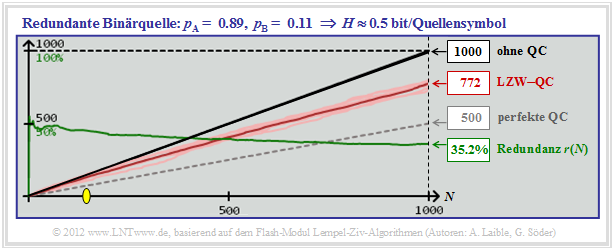LZW-Rest redundancy  $r(N)$  with redundant binary source  $(H = 0.5)$

.

$\text{Example 7:}$  The graphic shows simulations with  $N = 1000$  binary symbols.

• After averaging over ten series of experiments the result is  $r(N = 1000) ≈35.2\%$.
• below the yellow dot  $($in the example with  $N ≈ 150)$  the LZW algorithm even brings a deterioration.
• In this range, namely  $L > N$, that is:
The red curve is above the black one.

In the table below, the results for this redundant binary source  $(H = 0.5)$  are summarized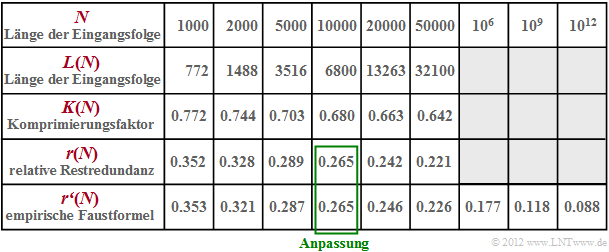Some numerical values for the efficiency of LZW coding
• The compression factor  $K(n)= L(n)/N$  decreases with increasing  $N$  only very slowly  (line 3).
• In line 4 the rest redundancy  $r(N)$  is given for different lengths between  $N =1000$  and  $N =50000$ ;
• According to relevant literature this residual redundancy decreases proportionally to  $\big[\hspace{0.05cm}\lg(N)\hspace{0.05cm}\big]^{-1}$ .
• In line 5 the results of an empirical formula are entered $($adaptation for $N = 10000)$:
$$r\hspace{0.05cm}'(N) = \frac{A}{ {\rm lg}\hspace{0.1cm}(N)}\hspace{0.5cm}{\rm with}$$
$$A = {r(N = 10000)} \cdot { {\rm lg}\hspace{0.1cm}10000} = 0.265 \cdot 4 = 1.06 \hspace{0.05cm}.$$
• You can see the good agreement between our simulation results  $r(N)$  and the rule of thumb  $r\hspace{0.05cm}′(N)$.
• You can also see that the residual redundancy of the LZW algorithm for  $N = 10^{12}$  is still  $8.8\%$ .
• For other sources, with other  $A$–values you will get similar results.  The principle process remains the same.  See also  Exercise 2.5  and  Task 2.5Z.